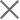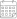Subscribe

### Indexes

Quarter 3-4, 2021 | Energy Market Review24 May 2022

In the third and the fourth quarters of 2021, Georgian power plants generated 3,766 mln. and 3,479 mln. kWh of electricity, respectively. This represents a 27.3% and 34.1% increase in total generation compared to the corresponding periods of the previous year (in 2020, the total generation in Q3 was 2,958 mln. kWh and 2,594 mln. kWh in Q4). On an annual basis, the increase in generation in the third quarter of 2021 was derived from a 30.8% and 27.4% increase in thermal and hydropower generation, respectively, more than offsetting the 8.5% decrease in wind power generation. The increase in production in the fourth quarter is related to the 3.4% and 63.7% increase in wind and hydropower generation, more than offsetting the 16.5% decrease in thermal power generation.

In the third and fourth quarters of 2021, electricity consumption on the local market amounted to 3,415 mln. (Q3) and 3,410 mln. kWh (Q4) (+20.9% and +17% compared to the third and fourth quarters of 2020, respectively) (Figure 1). Total power generation exceeded consumption by 351 mln.kWh and 69 mln.kWh in the third and the fourth quarters of the year, respectively. In percentage terms, in Q3 and Q4 there was a generation surplus amounting to 9.3% and 2% of total generation, respectively. In 2020 total generation exceeded consumption in the third quarter by 134 mln. kWh, around 4.5% of the total generation, and the difference between total generation consumption resulted in a shortage in the fourth quarter of 322 mln. kWh, around 12.4% of the total generation.